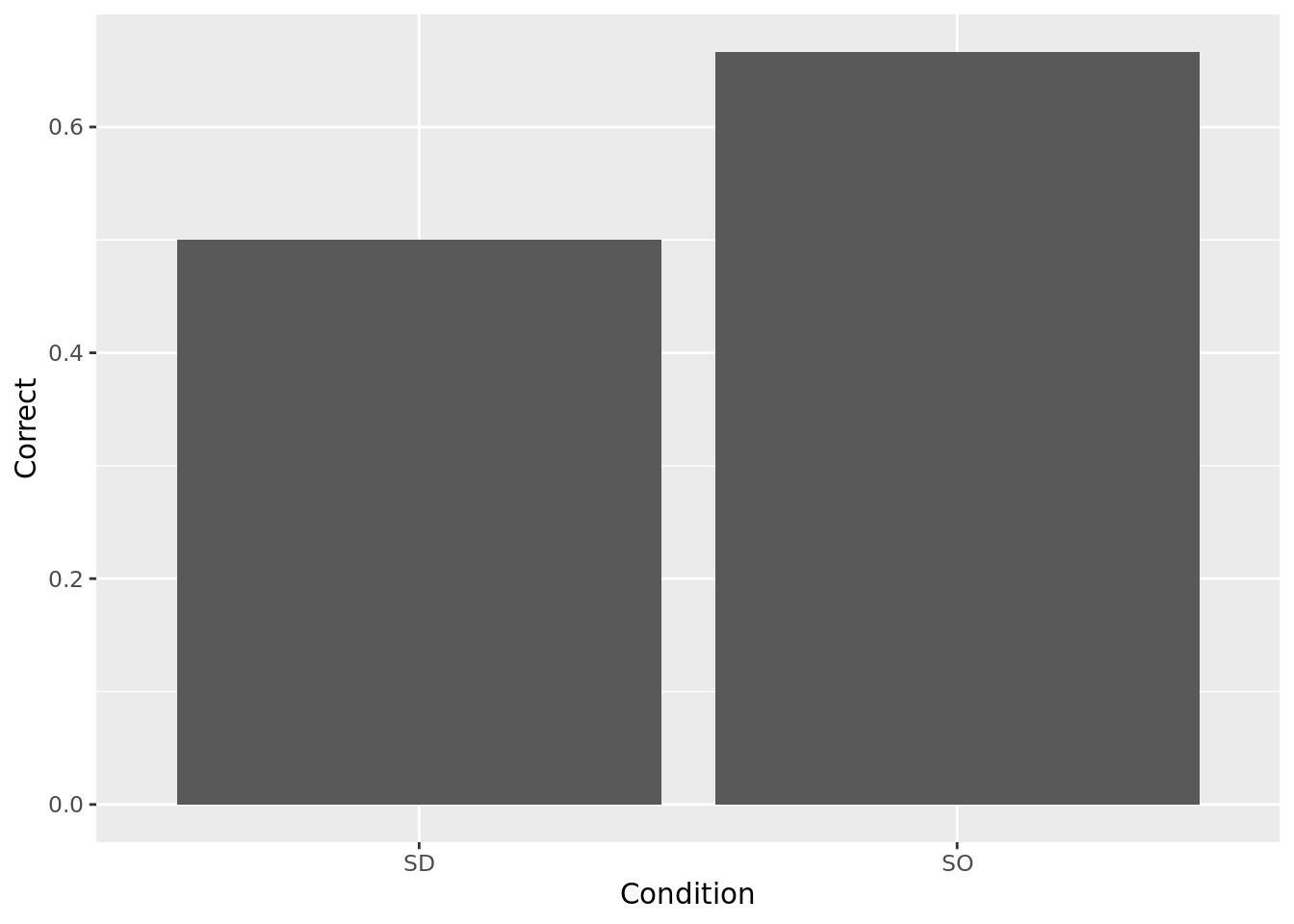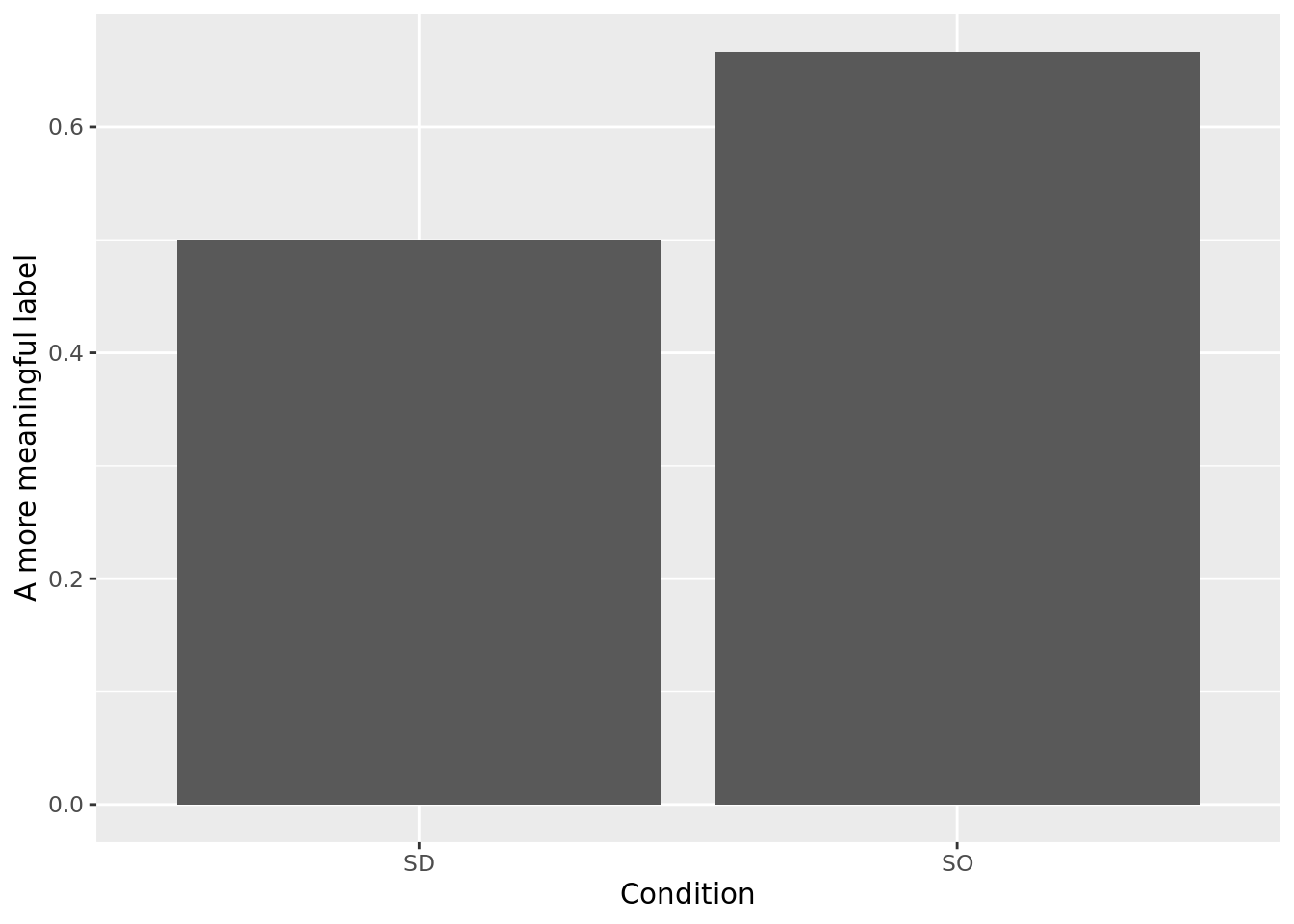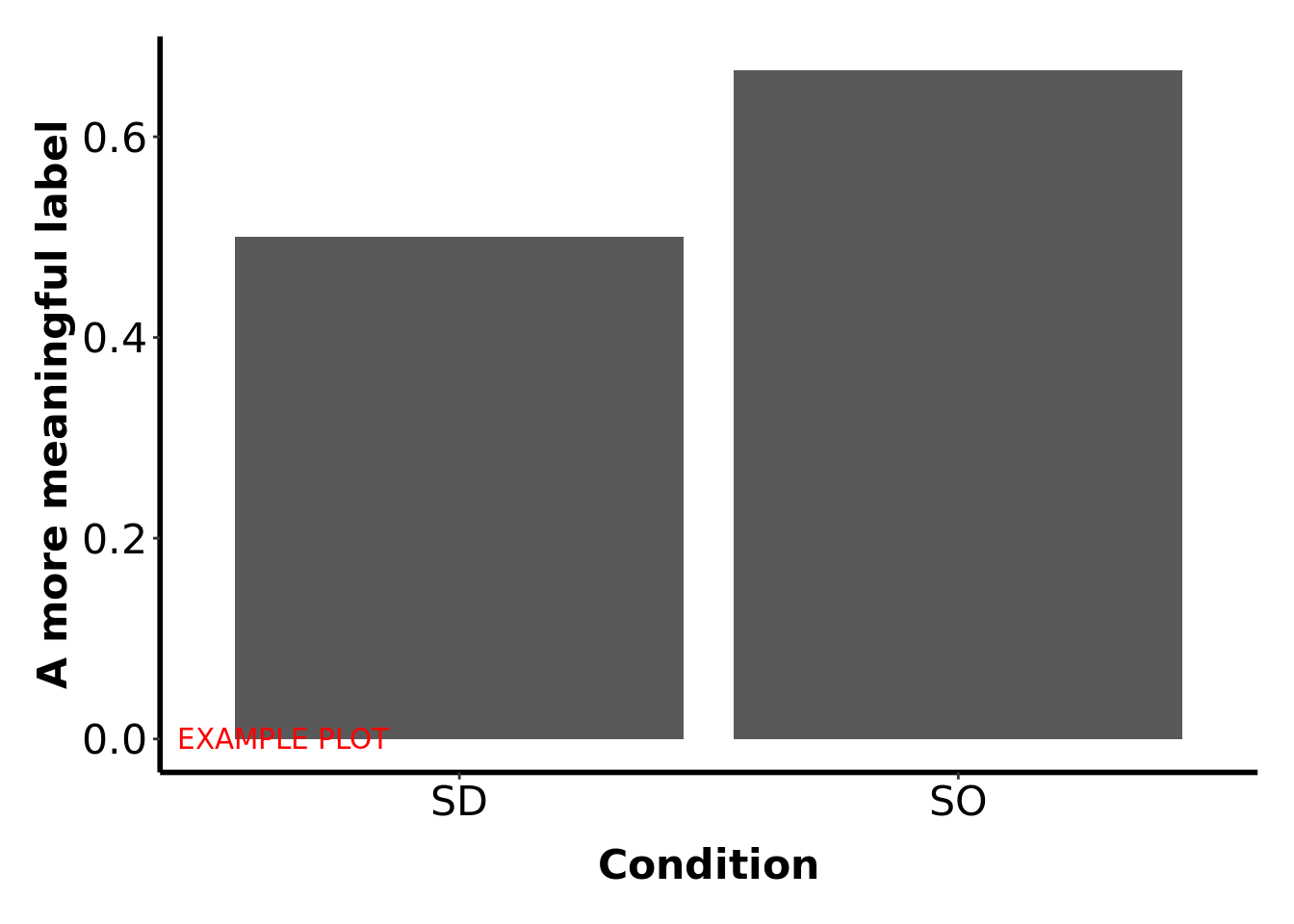## Before you start…

Before starting this exercise, you should have completed all the Absolute Beginners’, Part 1 workshop exercises. If not, take a look at those exercises before continuing. Each section below also indicates which of the earlier worksheets are relevant.

## Getting the data into R

Relevant worksheet: Intro to RStudio

In order to complete this worksheet, you’ll need to have downloaded your CSV file from the PsycEL exercise. See the instructions on PsycEL for how to do this.

Plymouth University students: Create/open your project named psyc412; within that create a script file called face-recog.R. Enter all commands into that script and run them from there.

Relevant worksheet: Exploring data

Load the tidyverse package, and load your data. Note: Everyone’s CSV file has a different name. For example, yours might be called 10435678 trials.csv. In the example below, you’ll need to replace facerec.csv with the name of your personal CSV file.

library(tidyverse)
recog <- read_csv("facerec.csv")

### Inspect

Look at the data by clicking on it in the Environment tab in RStudio. Each row is one trial of the final test. Here’s what each of the columns in the data set contain:

Column Description Values
TrialNum Trial number 0 - 47
Stimulus Picture shown on this test trial. Each picture has a unique code that identifies it. The number identifies the person, and the letter identifies which photograph it is of that person. e.g. 23B, 4A
Condition Type of picture shown on this test trial NS = person not shown during the study phase; SO = person shown during study, same photo; SD = person shown during study, different photo
Correct Did you get this trial correct? 1 = Yes, 0 = No

### How accurate were you?

Relevant worksheet: Exploring data.

Calculate your overall accuracy in this task – the proportion of trials you got right.The Correct column codes a correct response as 1 and an incorrect response as 0. This is helpful, because the mean of this column gives the proportion of trials you got right.

recog %>% summarise(mean(Correct))
# A tibble: 1 x 1
mean(Correct)
<dbl>
1           0.583

Your output will look something like the above, but the actual number won’t be the same. Enter the answer calculated from your own data in to your lab book.

### Old faces only

Relevant worksheet: Group differences.

These kinds of experiments typically focus on the faces that were presented during training (the “old” faces), so we’re going to filter out the trials on which you were shown a new person – the NS trials.

We have to tell the filter which trials we want to keep, so the filter we need is Condition != 'NS' where != means ‘not equal to’. We put this filtered data into a new data frame, which we’ve called recog.old (because it only contains “old” faces, i.e. the ones seen during training).

recog.old <- recog %>% filter(Condition != "NS")

### Does having the same photograph help?

Relevant worksheet: Group differences.

Were you more accurate when the photograph was the same as the one you studied, than when it was a different photograph of the same person? To find out, we group_by the type of trial (Condition) and then calculate the mean score for each group.

grpmeans <- recog.old %>% group_by(Condition) %>% summarise(Correct = mean(Correct))

We’ve put our answers into a new data frame, grpmeans, so go to the Enviornment window of RStudio and click on grpmeans’ to see your answers.

As before, you can safely ignore the “ungrouping” message that you receive.

Notice that we used summarise(Correct = mean(Correct)), rather than just summarise(mean(Correct)). This causes that column of our data frame to be called Correct, which is less of a mouthful than mean(Correct). The graphing command we’ll use next only works if the columns have very simple names (a single word, no special characters like brackets, etc.)

### Making a bar chart

Relevant worksheet: Exploring data.

Next, let’s make those two numbers into a bar chart. We didn’t cover bar charts in the Absolute Beginners’ Guide, so here’s how to do one:

meanplot <- grpmeans %>% ggplot(aes(x = Condition, y = Correct)) + geom_col()
meanplotYour output will look a bit like the above, but the heights of the bars will probably be different.

#### Explanation of command

meanplot <- - This gives the bar chart a name (meanplot), so we can modify it later without having to retype everything.

ggplot is the command in R used to draw graphs

aes tells ggplot which columns of your data frame to use in the plot. In this case, we want ‘Condition’ on the x axis, and ‘Correct’ (i.e. proportion correct) on the y axis.

+ geom_col() says that the type of graph we want is a bar chart (col is short for columns, another word for bars).

meanplot - This tells R to display the graph that we have named meanplot.

### Labelling a chart

Relevant worksheet: Exploring data.

Next, give your bar chart a more appropriate label for the y-axis. “Correct” isn’t very informative, “Mean proprotion correct” would make more sense. So, we’ll use the ylab command to add this y-axis label.

meanplot <- meanplot + ylab("A more meaningful label")
meanplotIf you get it right, it’ll look something like this – but of course with your meaningful label, rather than the one you see here.

### APA style

Relevant worksheet: Exploring data.

The graph you now have is readable, but it doesn’t look much like the graphs you seen in journal articles. In the Exploring data worksheet, we saw that we could change the way graphs looked using themes; for example, theme_bw(). In this exercise, we’re going to use a theme that makes graphs look like they do in journal articles. It’s called theme_APA because it’s based on how the Amercian Psychological Association says graphs should look.

The APA theme is not part of any R package at the moment, but you can download it from my website like this:

source("http://www.willslab.org.uk/rminr/theme-apa.R")

Now all you need to do is add this theme to your graph, using + theme_APA()`. If you get it right, your graph will look something like this (without the words “example plot”, of course, and with your meaningful y-axis label):